Daryl Funk

•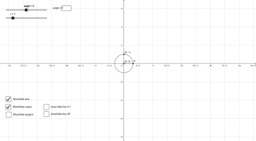Plotting cos, sin & tan as a function of angle in the circle

Activity

Daryl Funk

•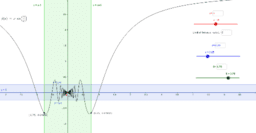epsilon-delta definition of a limit

Activity

Daryl Funk

•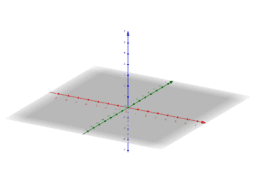Three planes

Activity

Daryl Funk

•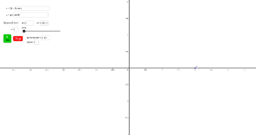Plotting parametric curves

Activity

Daryl Funk

•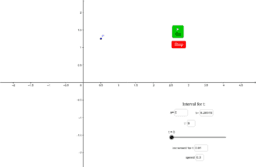Plotting parametric curves

Activity

Daryl Funk

•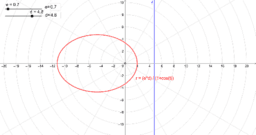Polar equations of conics

Activity

Daryl Funk

•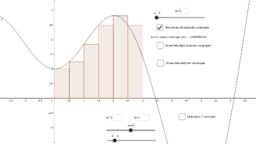Using Riemann Sums to approximate the area under a curve I

Activity

Daryl Funk

•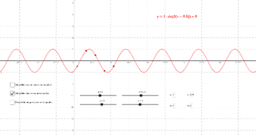Transformations of trig functions II

Activity

Daryl Funk

•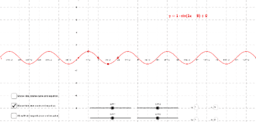Copy of Transformations of trig functions

Activity

Daryl Funk

•Plotting Polar Coordinates

Activity

Daryl Funk

•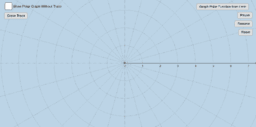Polar Function Graphing

Activity

Daryl Funk

•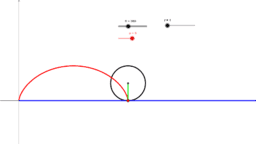The Cycloid

Activity

Daryl Funk

•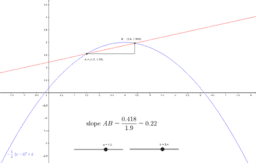Math 1120 Calculus I

Book

Daryl Funk

•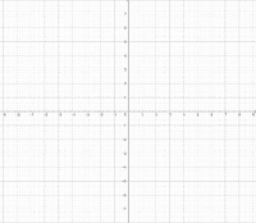some_implicit_curves

Activity

Daryl Funk

•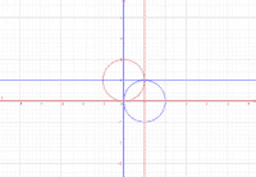A pair of orthogonal families of circles

Activity

Daryl Funk

•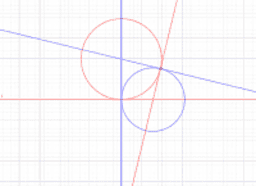A pair of orthogonal families of circles

Activity

Daryl Funk

•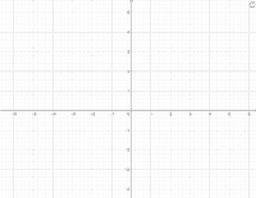some implicit curves

Activity

Daryl Funk

•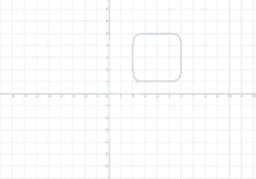(x-4)^6+(y-3)^6=64

Activity

Daryl Funk

•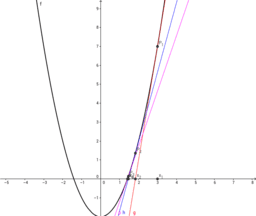Newton's Method for finding approximations to a root.

Activity

Daryl Funk

•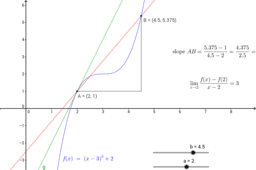Slope of a line tangent to a curve

Activity

Daryl Funk

•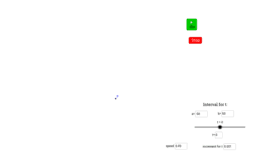The Butterfly Curve

Activity

Daryl Funk

•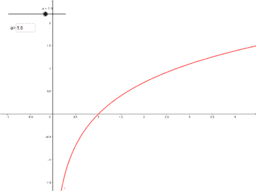Graphs of a function and its first few Taylor polynomials

Activity

Daryl Funk

•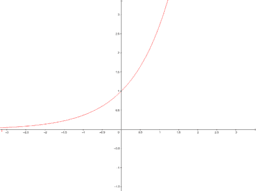Graphs of e^x and its first few Taylor polynomials

Activity

Daryl Funk

•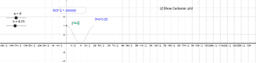Activity

Daryl Funk